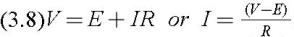Electric Motors and Drives: Fundamentals, Types and Applications, Third Edition

From the user's viewpoint the extent to which speed falls when load is applied, and the variation in speed with applied voltage are usually the first questions that need to be answered in order to assess the suitability of the motor for the job in hand. The information is usually conveyed in the form of the steady-state characteristics, which indicate how the motor behaves when any transient effects (caused for example by a sudden change in the load) have died away and conditions have once again become steady. Steady-state characteristics are usually much easier to predict than transient characteristics, and for the d.c. machine they can all be deduced from the simple equivalent circuit in Figure 3.6.

Under steady-state conditions, the armature current / is constant and equation (3.7) simplifies toThis equation allows us to find the current if we know the applied voltage, the speed (from which we get E via equation (3.6)) and the armature resistance, and we can then obtain the torque from equation (3.5). Alternatively, we may begin with torque and speed, and work out what voltage will be needed.

We will derive the steady-state torque-speed characteristics for any given armature voltage V in Section 3.4.3, but first we begin by establishing the relationship between the no-load speed and the armature voltage, since this is the foundation on which the speed control philosophy is based.

By no-load we mean that the motor is running light, so...### This is embarrasing...

An error occurred while processing the form. Please try again in a few minutes.